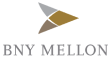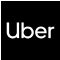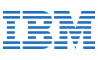New update is available. Click here to update.
Topics

# Sum Of Two Arrays

Easy0/40
Average time to solve is 15m+5 more companies

## Problem statement

You are given two numbers 'A' and 'B' in the form of two arrays (A[] and B[]) of lengths 'N' and 'M' respectively, where each array element represents a digit. You need to find the sum of these two numbers and return this sum in the form of an array.

Note:

``````1. The length of each array is greater than zero.

2. The first index of each array is the most significant digit of the number. For example, if the array A[] = {4, 5, 1}, then the integer represented by this array is 451 and array B[] = {3, 4, 5} so the sum will be 451 + 345 = 796. So you need to return {7, 9, 6}.

3. Both numbers do not have any leading zeros in them. And subsequently, the sum should not contain any leading zeros.
``````
Detailed explanation ( Input/output format, Notes, Images )
Constraints:
``````1 <= T <= 10^2
1 <= N, M <= 10^4
0 <= A[i], B[i] <= 9

Time Limit: 1 sec
``````
Sample Input 1:
``````2
4 1
1 2 3 4
6
3 2
1 2 3
9 9
``````
Sample Output 1:
``````1 2 4 0
2 2 2
``````
Explanation For Sample Input 1:
``````For the first test case, the integer represented by the first array is 1234 and the second array is 6, so the sum is 1234 + 6 =  1240.

For the second test case, the integer represented by the first array is 123 and the second array is 99, so the sum is 123 + 99 = 222.
``````
Sample Input 2:
``````2
3 3
4 5 1
3 4 5
2 2
1 1
1 2
``````
Sample Output 2:
``````7 9 6
2 3
``````Console